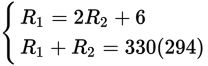# SAT Math Multiple Choice Question 489: Answer and Explanation

### Test Information

Question: 489

9.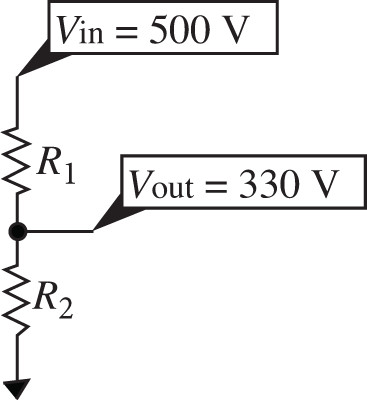A voltage divider is a simple circuit that converts a large voltage into a smaller one. The figure above shows a voltage divider that consists of two resistors that together have a total resistance of 294 ohms. To produce the desired voltage of 330 volts, R2 must be 6 ohms less than twice R1. Solving which of the following systems of equations gives the individual resistances for R1 and R2?

• A.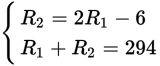• B.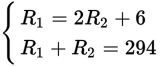• C.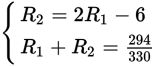• D.